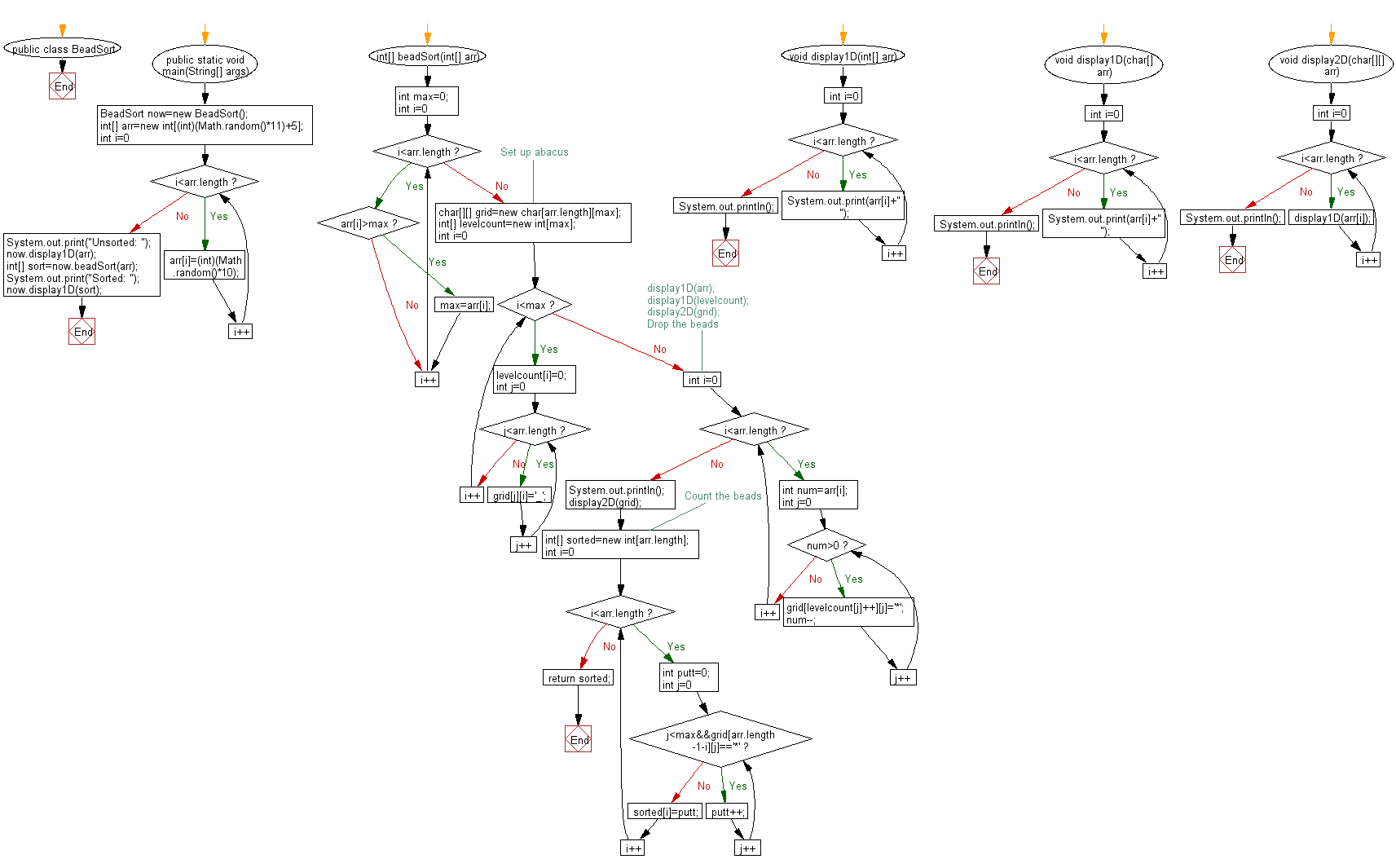﻿ Java exercises: Bead Sort Algorithm - w3resource# Java Exercises: Bead Sort Algorithm

## Java Sorting Algorithm: Exercise-8 with Solution

Write a Java program to sort an array of positive integers using the Bead Sort Algorithm.

According to Wikipedia "Bead sort, also called gravity sort, is a natural sorting algorithm, developed by Joshua J. Arulanandham, Cristian S. Calude and Michael J. Dinneen in 2002, and published in The Bulletin of the European Association for Theoretical Computer Science. Both digital and analog hardware implementations of bead sort can achieve a sorting time of O(n); however, the implementation of this algorithm tends to be significantly slower in software and can only be used to sort lists of positive integers. Also, it would seem that even in the best case, the algorithm requires O(n2) space".

Sample Solution:

Java Code:

``````public class BeadSort
{
public static void main(String[] args)
{
int[] arr=new int[(int)(Math.random()*11)+5];
for(int i=0;i<arr.length;i++)
arr[i]=(int)(Math.random()*10);
System.out.print("Unsorted: ");
now.display1D(arr);

System.out.print("Sorted: ");
now.display1D(sort);
}
{
int max=0;
for(int i=0;i<arr.length;i++)
if(arr[i]>max)
max=arr[i];

//Set up abacus
char[][] grid=new char[arr.length][max];
int[] levelcount=new int[max];
for(int i=0;i<max;i++)
{
levelcount[i]=0;
for(int j=0;j<arr.length;j++)
grid[j][i]='_';
}
/*
display1D(arr);
display1D(levelcount);
display2D(grid);
*/

for(int i=0;i<arr.length;i++)
{
int num=arr[i];
for(int j=0;num>0;j++)
{
grid[levelcount[j]++][j]='*';
num--;
}

}

System.out.println();
display2D(grid);
int[] sorted=new int[arr.length];
for(int i=0;i<arr.length;i++)
{
int putt=0;
for(int j=0;j<max&&grid[arr.length-1-i][j]=='*';j++)
putt++;
sorted[i]=putt;
}

return sorted;
}
void display1D(int[] arr)
{
for(int i=0;i<arr.length;i++)
System.out.print(arr[i]+" ");
System.out.println();
}
void display1D(char[] arr)
{
for(int i=0;i<arr.length;i++)
System.out.print(arr[i]+" ");
System.out.println();
}
void display2D(char[][] arr)
{
for(int i=0;i<arr.length;i++)
display1D(arr[i]);
System.out.println();
}
}
``````

Sample Output:

```Unsorted: 8 2 2 3 4 6 7 8 4 0 8

* * * * * * * *
* * * * * * * *
* * * * * * * *
* * * * * * * _
* * * * * * _ _
* * * * _ _ _ _
* * * * _ _ _ _
* * * _ _ _ _ _
* * _ _ _ _ _ _
* * _ _ _ _ _ _
_ _ _ _ _ _ _ _

Sorted: 0 2 2 3 4 4 6 7 8 8 8
```

Flowchart:Java Code Editor:

What is the difficulty level of this exercise?

﻿

## Java: Tips of the Day

Array vs ArrayLists:

The main difference between these two is that an Array is of fixed size so once you have created an Array you cannot change it but the ArrayList is not of fixed size. You can create instances of ArrayLists without specifying its size. So if you create such instances of an ArrayList without specifying its size Java will create an instance of an ArrayList of default size.

Once an ArrayList is full it re-sizes itself. In fact, an ArrayList is internally supported by an array. So when an ArrayList is resized it will slow down its performance a bit as the contents of the old Array must be copied to a new Array.

At the same time, it's compulsory to specify the size of an Array directly or indirectly while creating it. And also Arrays can store both primitives and objects while ArrayLists only can store objects.

Ref: https://bit.ly/3o8L2KH